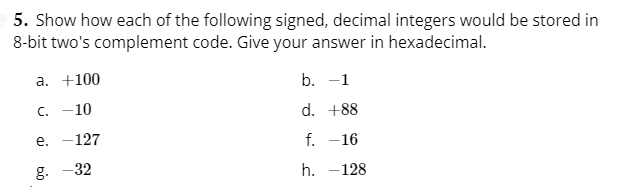# Question Solved1 Answer5. Show how each of the following signed, decimal integers would be stored in 8-bit two's complement code. Give your answer in hexadecimal. a. +100 b. -1 C. -10 d. +88 e. -127 f. -16 g. 32 h. -128F56QSK The Asker · Computer ScienceTranscribed Image Text: 5. Show how each of the following signed, decimal integers would be stored in 8-bit two's complement code. Give your answer in hexadecimal. a. +100 b. -1 C. -10 d. +88 e. -127 f. -16 g. 32 h. -128
More
Transcribed Image Text: 5. Show how each of the following signed, decimal integers would be stored in 8-bit two's complement code. Give your answer in hexadecimal. a. +100 b. -1 C. -10 d. +88 e. -127 f. -16 g. 32 h. -128